C语言中函数分为库函数和自定义函数。 库函数是C语言本身提供的函数。 ::: tip 通过http://www.cplusplus.com网站可查询到具体用法。 :::

##### 库函数举例
###### strcpy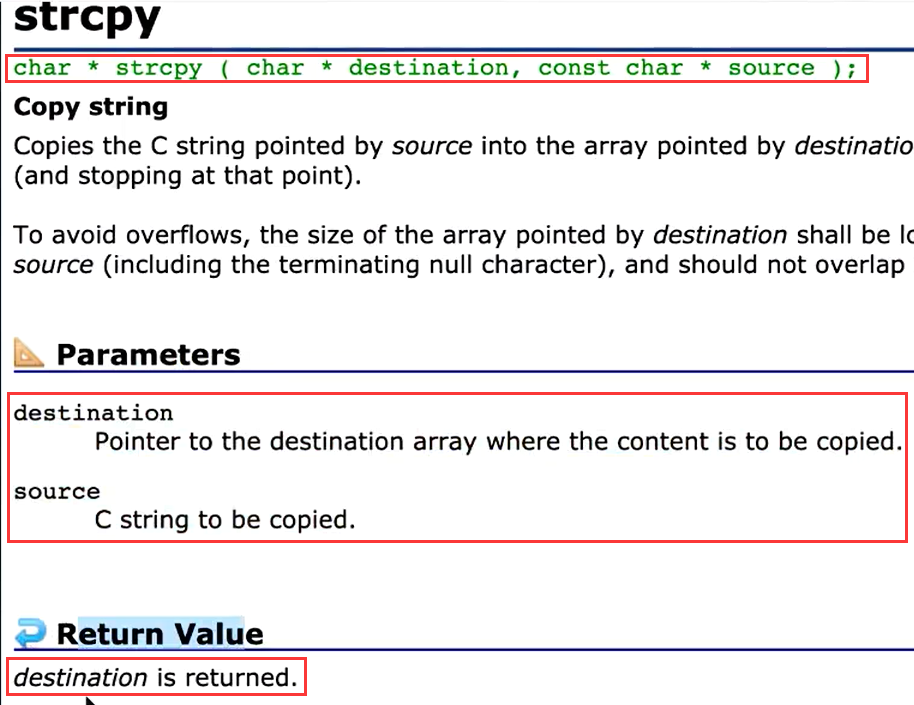``````#include <stdio.h>

int main()
{
char arr1[] = "Hello!";
char arr2 = "#############";
strcpy(arr2, arr1);
printf("%s", arr2);
return 0;
}``````
``Hello!``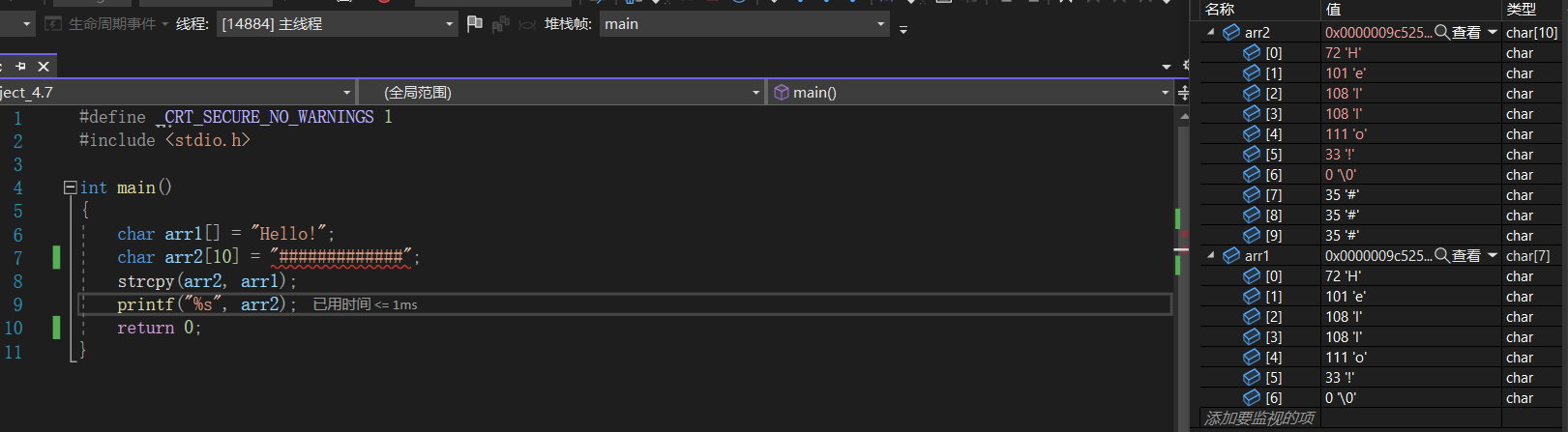由上图可得，函数strcpy拷贝数组时候，会将字符串的结束标志“\0”也拷贝过去。 ::: tip “\0”是字符串的结束标志。 :::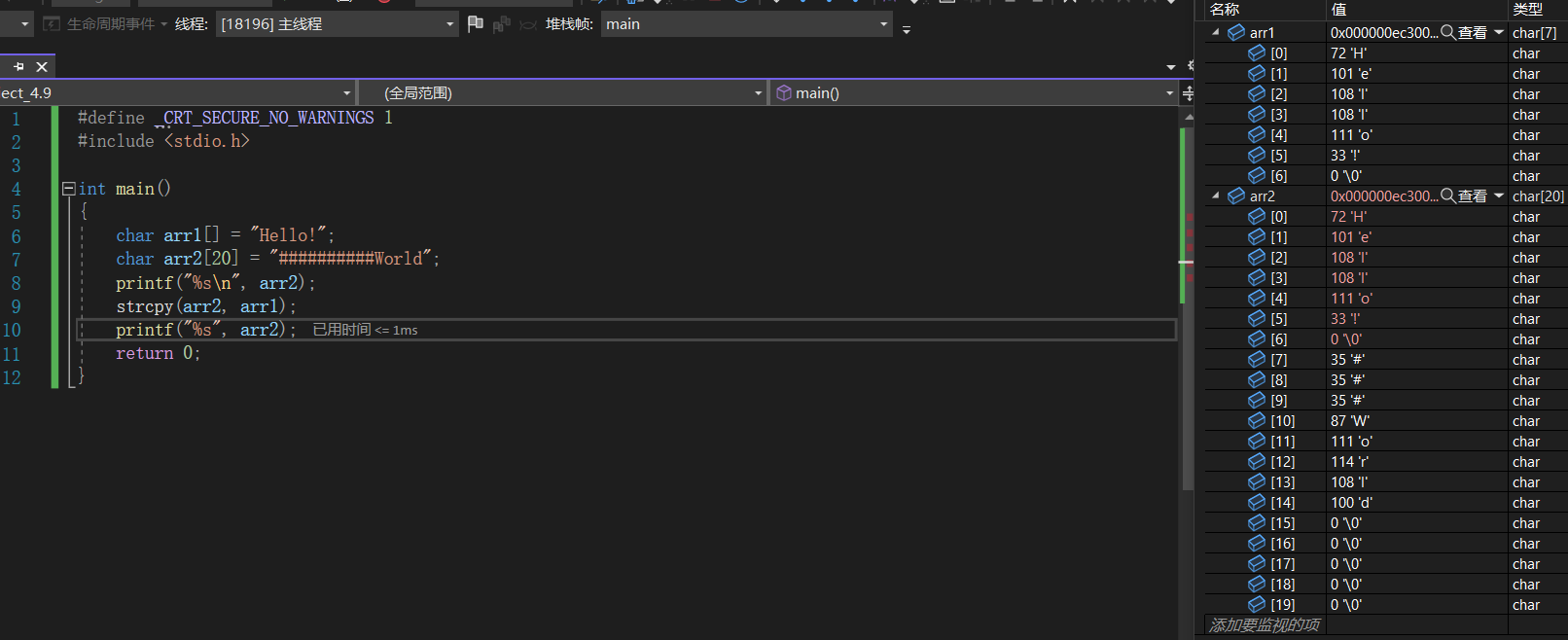上述代码中，如果没有把“\0”拷贝到arr2中，arr2中原有的“World”就会输出出来。

###### memset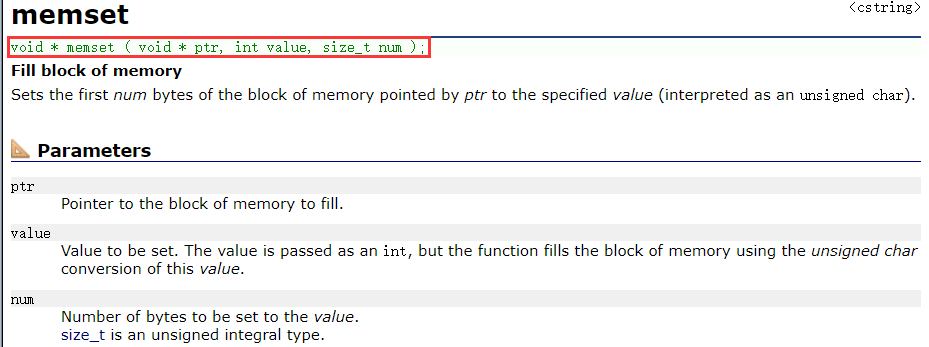函数menset的作用是将指针ptr指向的内存块的前num字节设置为指定值

``````#include <stdio.h>

int main()
{
char arr[] = "Hello World!";
memset(arr, '*', 5);
printf("%s\n", arr);
return 0;
}``````
``***** World!``

##### 自定义函数

``````#include <stdio.h>

int get_max(int x, int y)
{
if (x > y)
return x;
else
return y;
}

int main()
{
int a = 10;
int b = 20;
int max = get_max(a, b);
printf("max=%d\n", max);
max = get_max(500, 10);
printf("max=%d\n", max);
return 0;
}``````
``````max=20
max=500``````

::: tip 代码思路： 先写主函数，针对比较大小的需求封装函数get_max();将a和b两个参数传进去，get_max(a,b);，返回值给到变量max，然后可以输出。 定义函数时先写出get_max()，函数需要传进去a和b两个变量值，就需要有两个参数接受，格式是整型，使用int x,和int y，即get_max(int x,int y)。函数体中，判断大小后，return返回大的值。 :::

###### 写交换变量值的函数
``````#include <stdio.h>

void swap1(int x, int y)
{
int z;
z = x;
x = y;
y = z;
}

int main()
{
int a = 10;
int b = 20;
printf("a=%d b=%d\n", a, b);
swap1(a, b);
printf("a=%d b=%d", a, b);
return 0;
}``````
``````a=10 b=20
a=10 b=20``````

::: warning 通过debug调试可以看到上述的程序中，在函数swap中参数值交换后，并没有传到主函数中，没有改变主函数中参数的值。 x和y在内存中有独立的空间，和a、b并无关系。 :::

``````#include <stdio.h>

void swap2(int* pa, int* pb)  //不需要返回值，选择void类型
{
int z;
z = *pa;
*pa = *pb;
*pb = z;
}

int main()
{
int a = 10;
int b = 20;
printf("a=%d b=%d\n", a, b);
swap2(&a, &b);
printf("a=%d b=%d", a, b);
return 0;
}``````
``````a=10 b=20
a=20 b=10``````

::: tip 上述函数中，主函数中将a和b的地址传到swap函数中，swap函数中需要使用指针变量来接收传入的地址，即int* pa, int* pb。 pa中存放a的地址，pb中存放b的地址，pa、pb前加上，表示解引用，作用是通过地址找到所指向的内容，即pa = a，*pb = b。如下：

``````int main()
{
int a = 10;
int* pa = &a;
*pa = 20;
printf("a = %d", a);
}``````
``a = 20``

:::

##### 函数调用
``````#include <stdio.h>

void swap1(int x, int y)
{
int z = 0;
z = x;
x = y;
y = z;
}

void swap2(int* pa, int* pb)
{
int z = 0;
z = *pa;
*pa = *pb;
*pb = z;
}

int main()
{
int a = 10;
int b = 20;
swap1(a, b);
printf("a=%d,b=%d\n", a, b);
swap2(&a, &b);
printf("a=%d,b=%d", a, b);
return 0;
}``````
``````a=10,b=20  //swap1调用后
a=20,b=10  //swap2调用后``````

##### 实例
###### 列出100到200之间的素数

``````#include <stdio.h>

int main()
{
int a = 10;
int isPrime = 0;
for (a = 100;  a < 200; a++){
int i = 0;
int isPrime = 1;
for (i = 2; i < a; i++){
if (a % i == 0){
isPrime = 0;
break;
}
}
if (isPrime == 1) {
printf("%d\n", a);
}
}
return 0;
}``````

``````#include <stdio.h>

int isPrime(int n) //使用形参n接收主函数传来的实参i
{
int j = 0;
for (j = 2; j < n; j++)  //判断条件可以改为j <= sqrt(n)来优化程序（添加头文件#include <math.h>）
{
if (n % j == 0)
{
return 0;
//比break的作用更强，break只能跳出当前循环，return0可以结束整个函数
//return0后直接跳到24行，即：有一个约数就证明不是素数，停止isPrime循环
}
}
return 1;  //当j从2到n-1都试过不可以整除n时，即n为素数，此时j=n，跳出for循环，返回1
}
int main()
{
//打印100-200之间的素数
int i = 0;
for (i = 100; i <= 200; i++)
{
if (isPrime(i) == 1)
//规定i为素数时isPrime返回1，反之为0
{
printf("%d ", i);
}
}
return 0;
}``````
``101 103 107 109 113 127 131 137 139 149 151 157 163 167 173 179 181 191 193 197 199``

::: warning 上述函数中不可以在自定义函数isPrime中的for循环内过早的使用if和else来选择return0还是return1，原因是此时只判断了数字2是否能被整除，并没有判断到n-1。 :::

###### 计算闰年
``````#include <stdio.h>

int is_leap_year(int a)
{
if ((a % 4 == 0 && a / 100 != 0) || a % 400 == 0)
{
return 1;
}
else
{
return 0;
}
}

int main()
{
int year = 0;
for (year = 2000; year <= 2020; year++)
{
if (is_leap_year(year) == 1)
{
printf("%d " ,year);
}
}
}``````
``2000 2004 2008 2012 2016 2020``

::: warning 自定义函数中一般不做输出，只完成纯粹的功能如上述代码判断闰年，是返回1不是返回0，主函数中根据自定义函数的返回值来写条件判断输出语句。 单一功能原则，增加了代码复用性。 :::

###### 整形有序数组二分查找
``````#include <stdio.h>

int binary_search(int m, int n, int arr1[])
{
int left = 0;  //最左边元素下标
int right = n - 1;  //最右边元素下标
while (left <= right)  //左右下标相等表示锁定某一个元素，还需要进入循环判断是否等于m
{
int mid = (left + right) / 2;  //中间元素的下标
if (m < arr1[mid])
{
right = mid - 1;
}
else if (m > arr1[mid])
{
left = mid + 1;
}
else
{
return mid;
}
}
return -1;
}

int main()
{
int arr[] = { 1,2,3,4,5,6,7,8,9 };
int sz = sizeof(arr) / sizeof(arr);
int a = 7;
int b = binary_search(a, sz, arr);
if (b == -1)
{
printf("没有找到");
}
else
{
printf("找到了，在第%d位",b);
}
return 0;
}``````
``找到了，在第6位``

::: warning 上述代码第9行注意不要写在while循环外，否则变量mid不会改变。 上述代码第32行，写成“=”是赋值，写成“==”是判断。 ::: ::: warning 形参是实参的一部分临时拷贝。但数组传参时候，为了避免空间浪费，传过去的只是数组第一个元素的地址，即自定义函数中数组名代表首元素的地址自定义函数中的arr1[]用来接收主函数中数组的首元素地址，本质上是一个指针。 上述代码在vs2022中调试可以看到，自定义函数中数组arr1内只有一个元素“1”。 :::

::: danger sizeof不能用来计算指针大小。 原因如下： sizeof(指针)：返回计算机系统的地址字节数，如果是64为系统，返回8；32位系统，返回4；16位系统，返回2。 sizeof(arr1)第一个元素是int类型，大小是4。 两个相除的结果是2或1或0。 ::: ::: danger 函数内部求参数部分数组元素个数是做不到的。 上述代码中第29行放在函数binary_search中无法求出正确结果。 解决方法是在函数外面计算出数组结果后再传递进去。 :::

###### 写一个函数，每次调用函数后，num值+1
``````#include <stdio.h>

{
(*p)++;  //“*p”即主函数中的num。
//“++”的优先级高于指针“* ”，“* p”需要使用括号
}

int main()
{
int num = 0;
printf("num=%d\n", num);  //1
printf("num=%d\n", num);  //2
printf("num=%d\n", num);  //3
return 0;
}``````

::: tip 函数内部改变num变量值（函数内操作函数外的变量，需要使用“传址”的方式） :::

##### 函数嵌套调用和链式访问
###### 函数嵌套调用

``````#include <stdio.h>

Printf()
{
printf("hello world\n");
}

PRINTF()
{
int i = 0;
for (i; i < 3; i++)
{
Printf();
}
}

int main()
{
int num = 0;
PRINTF();
return 0;
}``````
``````hello world
hello world
hello world``````
###### 函数链式访问

``````#include <stdio.h>

int main()
{
int len = 0;
len = strlen("abc");
printf("%d\n", len);
printf("%d\n", strlen("abc"));  函数strlen的返回值做函数printf的参数
return 0;
}``````
``````3
3``````

###### 举例
``````#include <stdio.h>
int main()
{
printf("%d", printf("%d", printf("%d", 43)));
return 0;
}``````
``4321``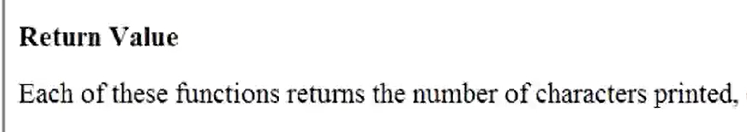::: tip 上图printf返回值的介绍中，每一个这样的函数返回的都是打印字符的个数。 上述程序最内层的printf函数打印的内容为“43”，共两个字符，即最内层的printf函数的返回值为2。从内向外第二层printf打印的内容为“2”，共一个字符，即从内向外第二层printf的返回值为1，最外层printf打印的内容“1”。 即第4行代码共打印内容为“4321”。 :::

##### 函数声明和定义
###### 函数声明

``````#include <stdio.h>

int main()
{
int a = 10;
int b = 20;
int c = Add(a, b);  //函数调用
printf("%d", c);
return 0;
}

int Add(int x, int y)  //函数定义（函数的具体实现，交代函数功能实现）
{
int z = x + y;
return z;
}``````

::: warning 头文件中放函数声明，源文件中放函数定义。 ::: ::: tip 在VS中，新建的头文件.h和源文件.c构成一个模块，如下述程序的“加法模块”。 需要使用该“加法模块”时，在主函数所在的文件中主函数上面，添加【#include "add.h"】就可以了。（引用自己写的头文件使用双引号，引用函数库中的头文件使用尖括号。） :::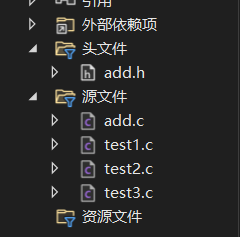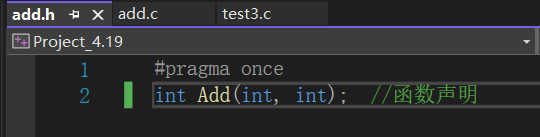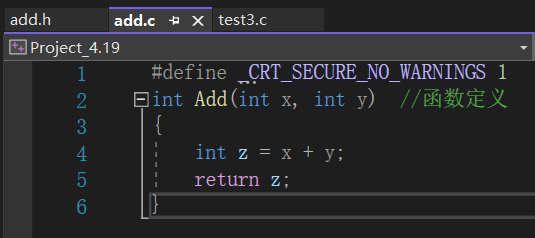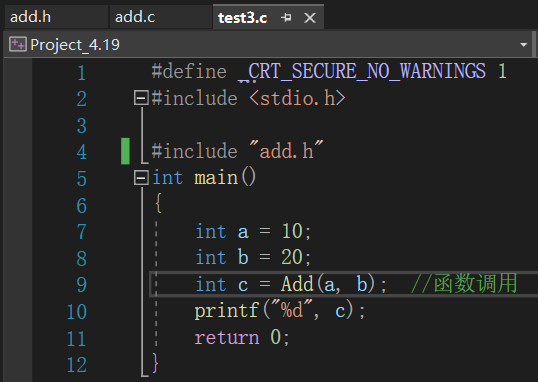功能太过复杂可以使用分模块写法：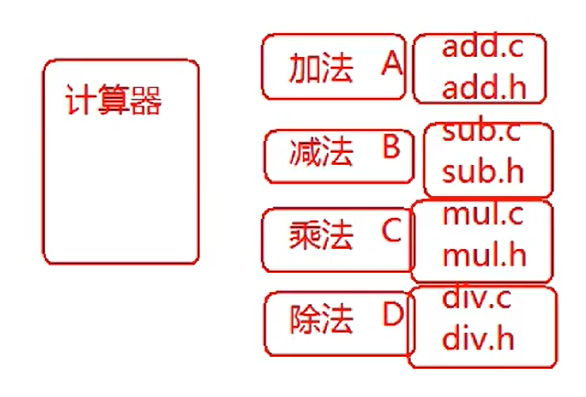::: tip 在正规工程中，上述计算机的各个模块需要使用时，写一个.h和.c文件，把函数声明放在.h文件中，把函数的定义放在.c文件中，在需要用的文件中引用头文件即可。（函数先声明后使用） :::Suzhou
Lv1

17

9

0